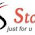48 SMART: Return On Equity

Thursday, 12 April 2012

Return On Equity

Return On Equity

Do you know what is Return on equity (R.O.E)?
As a fresh guy in investment knowledge, I'm would like to share what i know about R.O.E.
Actually i knew this word came from Warren Buffett book. R.O.E is one of the main consider factors on picking up potential profitable company in stock market.

This has made me feel interesting and knee to know about what is R.O.E. Eventually i search the info about ROE in internet, but i found it is very difficult to understand for me as newbie.

I try to simplify what i can understand and shares with public.

ROE is measuring how great a company can generate great income from existing assets. Somebody declare ROE is a gauge of profit-generating efficiency.
HIGH ROE represent the company is good of handling their asset and earn money for stock holder.

For example,

Company A use RM1.00 of modal earn RM0.20 every year. ROE = 0.20.
Company B use RM1.00 of modal earn RM0.50 every year. ROE = 0.50.

This show that company B is good of handling their modal and earn as much as they can. But company A only can earn RM0.20 less compare with company B. which their modal are same of RM1.00.

Many investors invests in companies that generate profits more efficient than their rivals with measuring the ROE. Of course, this is not the only factor need to consider for investors.

Investopedia explains 'Return On Equity - ROE'

The ROE is useful for comparing the profitability of a company to that of other firms in the same industry.

There are several variations on the formula that investors may use:

1. Investors wishing to see the return on common equity may modify the formula above by subtracting preferred dividends from net income and subtracting preferred equity from shareholders' equity, giving the following: return on common equity (ROCE) = net income - preferred dividends / common equity.

2. Return on equity may also be calculated by dividing net income by average shareholders' equity. Average shareholders' equity is calculated by adding the shareholders' equity at the beginning of a period to the shareholders' equity at period's end and dividing the result by two.

3. Investors may also calculate the change in ROE for a period by first using the shareholders' equity figure from the beginning of a period as a denominator to determine the beginning ROE. Then, the end-of-period shareholders' equity can be used as the denominator to determine the ending ROE. Calculating both beginning and ending ROEs allows an investor to determine the change in profitability over the period.

1 comment:

1.This comment has been removed by a blog administrator.